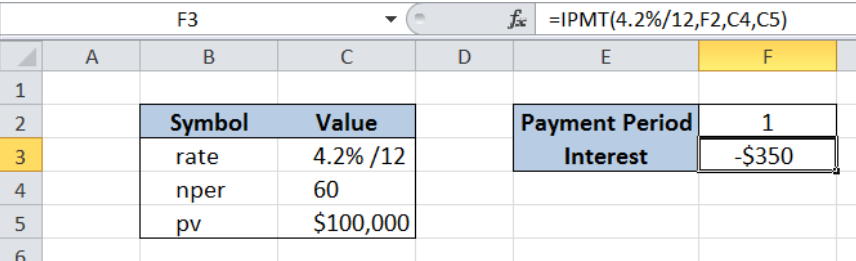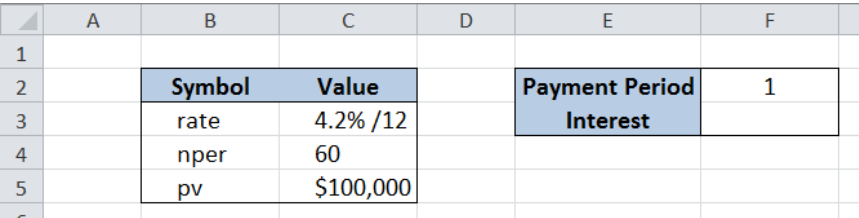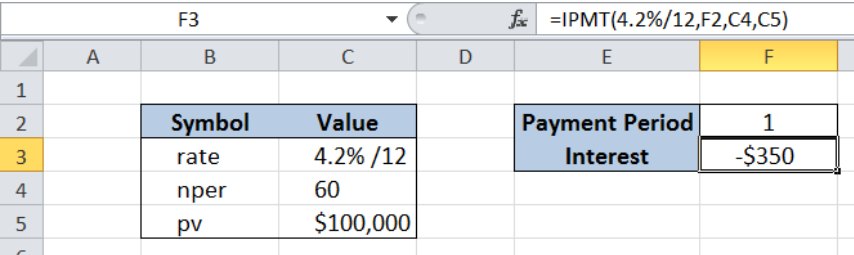Get instant live expert help with Excel or Google Sheets“My Excelchat expert helped me in less than 20 minutes, saving me what would have been 5 hours of work!”

#### Post your problem and you’ll get Expert help in seconds.

Your message must be at least 40 characters
Our professional Expert are available now. Your privacy is guaranteed.

# Calculate interest for given period

Excel allows us to calculate the interest payment for any loan using the IPMT function. This step by step tutorial will assist all levels of Excel users in calculating the interest paid for a given period.Figure 1. Final result: Calculate interest for given period using IPMT function

Final formula: `=IPMT(4.2%/12,F2,C4,C5)`

## Syntax of the IPMT Function

IPMT returns the interest paid in a given period for any investment or loan

`=IPMT(rate, per, nper, pv, [fv], [type])`

where

• rate – the interest rate for a period of payment; if payment is monthly, rate should be annual rate divided by 12
• per – payment period for the interest payment we want to calculate
• npertotal number of payment periods
• pv – the present value of a loan
• [fv]optional; the future value of a loan after the last payment; if omitted, the default value is 0
• [type] –   optional; specifies the timing of the payment
• 0 (zero) – payment at the end of the period; also the default value
• 1 – payment at the start of the period

## Setting up the Data

Our table consists of two columns: Symbol (column B) and Value (column C).  We input the values in column C for the interest rate (4.2% annually), total number of payments (60 months) and loaned amount (\$100,000).

In cell F2, we enter the payment period for which we want to calculate the interest paid, which is 1, or the 1st month.  The interest paid on the first month of payment will be recorded in cell F3.Figure 2. Sample data for the IPMT formula

## Calculate interest paid on first month

We want to determine the interest paid on the first month of payment for a five-year loan of \$100,000, with an annual interest rate of 4.2%.  In order to calculate the interest using IPMT function, we follow these steps:

Step 1.  Select cell F3

Step 2.  Enter the formula: `=``IPMT(4.2%/12,F2,C4,C5)`

Step 3.  Press Enter

Since we make monthly payments, the annual interest rate 4.2% is divided by 12 to get the monthly rate.  The period is 1, which stands for the first month of payment.  The total number of periods is 60 because 5 years is equivalent to 60 months.  The present loan value is \$100,000.

As a result, the interest calculated in cell F3 is  -\$ 350.  It is a negative value because it is an outgoing cash flow, or payment.Figure 3. Entering the IPMT formula to calculate the interest for the first month

Most of the time, the problem you will need to solve will be more complex than a simple application of a formula or function. If you want to save hours of research and frustration, try our live Excelchat service! Our Excel Experts are available 24/7 to answer any Excel question you may have. We guarantee a connection within 30 seconds and a customized solution within 20 minutes.

### Did this post not answer your question? Get a solution from connecting with the expert.Another blog reader asked this question today on Excelchat:## Subscribe to Excelchat.coAnother blog reader asked this question today on Excelchat: